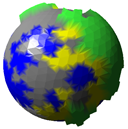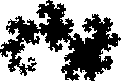20 Feb

For the last few days, I have been working on a planet generator. I originally got the idea from the method how you can easily tessellate sphere into triangles. This results in a spherical map instead of a cylindrical map.The usual way of rendering a planet as a a sphere with cylindrical texture map (or height field) looks quite bad in places because the map is stretched on the equator and pinched near the poles. The method described below takes a different approach and generates a map of vertices connected by triangles.

The vertices contain the terrain information such as terrain type (sea, land, mountain) and height. More detail is introduced by subdividing the triangles into smaller triangles.

The starting shape is an octahedron. We then subdivide each side into four triangles, normalize the new vertices and apply simple physics to move all the vertices away from the others (reverse gravity) – this eventually settles the vertices quite evenly (it won’t be a perfect shape but it is good enough for most purposes). We don’t even need to start from a symmetrical shape, using a naive repulsion algorithm eventually makes the shape symmetric as long as it is possible.

The number of subdivisions can be variable and dynamic. I’m planning to make the generator subdivide triangles on demand. In a game, you would see approximate shapes from the orbit but when flying close to the surface, the shorelines etc. would have a lot more detail. You can subdivide infinitely which means there will be infinite detail.Dragon curve

I use a very simple algorithm to decide the terrain type on each vertex, simply the first point of the triangle decides the terrain type on the new triangle. This results into the Dragon curve fractal. A more sophisticated algorithm would change the subdivision rules according to the subdivision depth. For example, the same rules shouldn’t apply when subdividing on a continental scale versus to a scale of a few kilometers.

Next, I’m going to model a very simple simulation of atmosphere including prevailing winds, cloud formation and temperature.